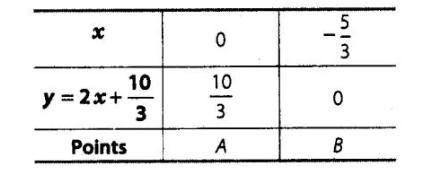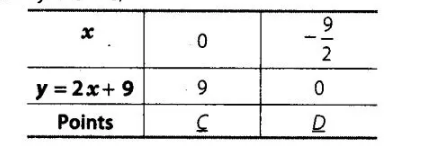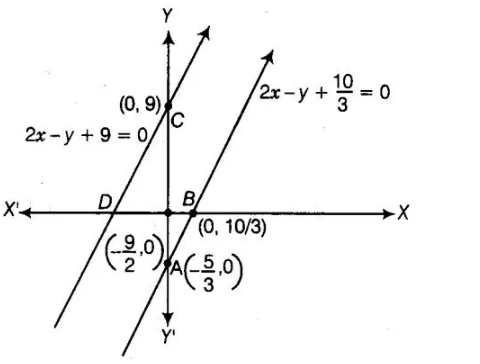# Graphically, the pair of equations

Question:

Graphically, the pair of equations

6x – 3y + 10 = 0

2x – y + 9 = 0

represents two lines which are

(a) intersecting at exactly one point

(b) intersecting exactly two points

(c) coincident

(d) parallel

Solution:

The given equations are

$6 x-3 y+10=0$

$\Rightarrow 2 x-y+\frac{10}{3}=0$ [dividing by 3 ]... (i)

and $\quad 2 x-y+9=0$  .......(ii)

Now, table for $2 x-y+\frac{10}{3}=0$,and table for $2 x-y+9=0$,Hence, the pair of equations represents two parallel lines.